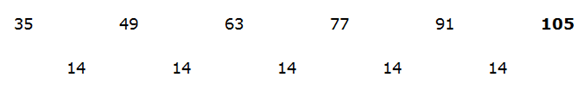# SBI Clerk Prelims 2021 Quantitative Aptitude Questions (Day-44)

Dear Aspirants, Our IBPS Guide team is providing new series of Quantitative Aptitude Questions for SBI Clerk Prelims 2020 so the aspirants can practice it on a daily basis. These questions are framed by our skilled experts after understanding your needs thoroughly. Aspirants can practice these new series questions daily to familiarize with the exact exam pattern and make your preparation effective.

Start Quiz

Missing number series

Directions (01-05): What value should come in the place of (?) in the following number series?

1) 4, 10, 33, 136, ?, 4116

A.680

B.685

C.720

D.785

E.815

2) 1944, 968, 480, 236, 114, ?

A.53

B.60

C.65

D.70

E.49

3) 35, 49, 63, 77, 91, ?

A.100

B.102

C.103

D.105

E.115

4) 88, 165, 220, 253, ?

A.261

B.258

C.264

D.268

E.270

5) 85, 110, 94, 103, 99, ?

A.20

B.60

C.80

D.100

E.110

Quantity based sums

Directions (06-10): Following questions have two quantities as Quantity I and Quantity II. You have to determine the relationship between them and give answer as,

6) Quantity I: What is the perimeter of the rectangle whose area is 216 cm2 and the ratio of length to breadth is 3: 2?

Quantity II: 63 cm

A.Quantity I > Quantity II

B.Quantity I ≥ Quantity II

C.Quantity I = Quantity II

D.Quantity I < Quantity II

E.Quantity I ≤ Quantity II

7) Quantity I:
Compound interest on Rs.1800 at 10% per annum for 2 years?

Quantity II: What is the difference between the simple and compound interest on Rs.37800 at 10% per annum for two years?

A.Quantity I > Quantity II

B.Quantity I ≥ Quantity II

C.Quantity I = Quantity II

D.Quantity I < Quantity II

E.Quantity I ≤ Quantity II

8) Quantity I:
18 seconds

Quantity II: Time taken by 180 m train crosses a man at the speed of 20 kmph.

A.Quantity I > Quantity II

B.Quantity I ≥ Quantity II

C.Quantity I = Quantity II

D.Quantity I < Quantity II

E.Quantity I ≤ Quantity II

9) Quantity I:
Ratio of the present age of A and B is 4: 5 and after 8 years the ratio becomes 5: 6. What is the difference between the ages of A and B after 15 years?

Quantity II: 6 years

A.Quantity I > Quantity II

B.Quantity I ≥ Quantity II

C.Quantity I = Quantity II

D.Quantity I < Quantity II

E.Quantity I ≤ Quantity II

10) Quantity I:
A and B can complete a work in 10 days and 18 days respectively. If C’s efficiency is 50% more than B, in how many days A, B and C together can complete the work?

Quantity II: 5 days

A.Quantity I > Quantity II

B.Quantity I ≥ Quantity II

C.Quantity I = Quantity II

D.Quantity I < Quantity II

E.Quantity I ≤ Quantity II

Answers :

1) Answer: B

4 * 2 + 2 = 10

10 * 3 + 3 = 33

33 * 4 + 4 = 136

136 * 5 + 5 = 685

685 * 6 + 6 = 4116

2) Answer: A

1944/2 – 4 = 968

968/2 – 4 = 480

480/2 – 4 = 236

236/2 – 4 = 114

114/2 – 4 = 53

3) Answer: D4) Answer: C

88 + 77 = 165

165 + 55 = 220

220 + 33 = 253

253 + 11 = 264

5) Answer: D

85 + 52 = 110

110 – 42 = 94

94 + 32 = 103

103 – 22 = 99

99 + 12 = 100

6) Answer: D

From Quantity I,

Area of the rectangle = 216

3x * 2x = 216

x= 36

x = 6 cm

Perimeter of the rectangle = 2 * (3 * 6 + 2 * 6) = 60 cm

From Quantity II,

63 cm

Quantity I < Quantity II

7) Answer: C

From Quantity II,

CI = P (1 + R/100)2 – P

CI = 1800 * (1 + 10/100)2 – 1800

= 378

From Quantity II,

Difference = 37800 * 10 * 10/(100 * 100)

= 378

Quantity I = Quantity II

8) Answer: D

From Quantity I,

18 seconds

From Quantity II,

Required time = 180/(20 * 5/18) = 32.4 seconds.

Quantity I < Quantity II

9) Answer: A

From Quantity I,

(4x + 8)/(5x + 8) = 5/6

25x + 40 = 24x + 48

x = 8 years

A = 4 * 8 = 32 years

B = 5 * 8 = 40 years

After 15 years A and B’s age is 47 and 55 years respectively.

Difference between the ages of A and B = 8 years

From Quantity II,

6 years

Quantity I > Quantity II

10) Answer: D

From Quantity I,

A = 1/10

B = 1/18

C = 18 * 100/150 = 12 days

A + B + C = 1/10 + 1/18 + 1/12

= (18 + 10 + 15)/180

= 43/180

Required time = 180/43 days

From Quantity II,

5 days

Quantity I < Quantity II

 Check Here to View SBI Clerk Prelims 2021 Quantitative Aptitude Questions Day – 43 Day – 42 Day – 41
3.3 3 votes
Rating0 Comments
Inline Feedbacks
View all comments